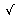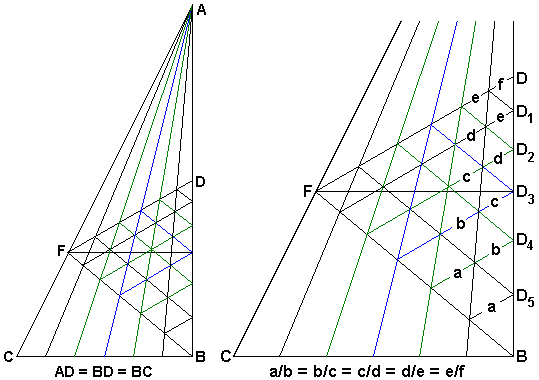# What Is Wrong? (Delian Problem Solved)

It's well known that the famous Delian problem admits no solution in the classical construction framework with ruler and compass.

However, consider the following applet.

### This applet requires Sun's Java VM 2 which your browser may perceive as a popup. Which it is not. If you want to see the applet work, visit Sun's website at https://www.java.com/en/download/index.jsp, download and install Java VM and enjoy the applet.

 What if applet does not run?

The main structure consists of a right triangle with the vertical side of length 2 and the horizontal side of length 1. Mark a point on the vertical side at the distance2 from the apex. Through that point draw a horizontal line to the intersection with the hypotenuse. Join the point of intersection to the midpoint and the foot of the vertical side. Divide the base into a number of equal segments (12 in the "real world" situation). The construction involves joining some points and drawing through some of the points of intersection lines parallel to other lines. The applet above presents some possible mesh configurations. Everything's nice and fits tightly.

My main interest lies with the configuration in which the base has been divided into six equal parts.By construction, AD = BD = BC = 1 and AD3 =2. The base BC is divided into 6 equal parts. The lines from A to the division points intersect DF and BF at five points each, from which lines are drawn parallel to BF and DF, respectively.

By considering pairs of triangles with bases parallel to DF and the apex at A, we derive a five term proportion:

 a/b = b/c = c/d = d/e = e/f.

The six small triangles that line up on BD have all three corresponding sides parallel and are, therefore, similar. The above proportion indicates the constant ratio, say r, in which the corresponding sides of any two adjacent triangles stand to each other. Let DD1 = m. Then D1D2 = mr, D2D3 = mr2, D3D4 = mr3, D4D5 = mr4, and, finally, D5B = mr5.

Summing all six up, we obtain m·(r6 - 1)/(r - 1) = 1. If we only add the first three terms, we see that m·(r3 - 1)/(r - 1) = DD3 =2 - 1. Now, divide the first identity by the second:

 (r6 - 1)/(r3 - 1) = 1/(2 - 1),

or

 r3 + 1 =2 + 1.

In other words, r3 =2, which is to say r2 = 21/3. The latter of course shows that the famous Delian problem of duplication of the cube is solvable with ruler and compass.

How is that possible? What is wrong? Sometimes you should not believe what you see.